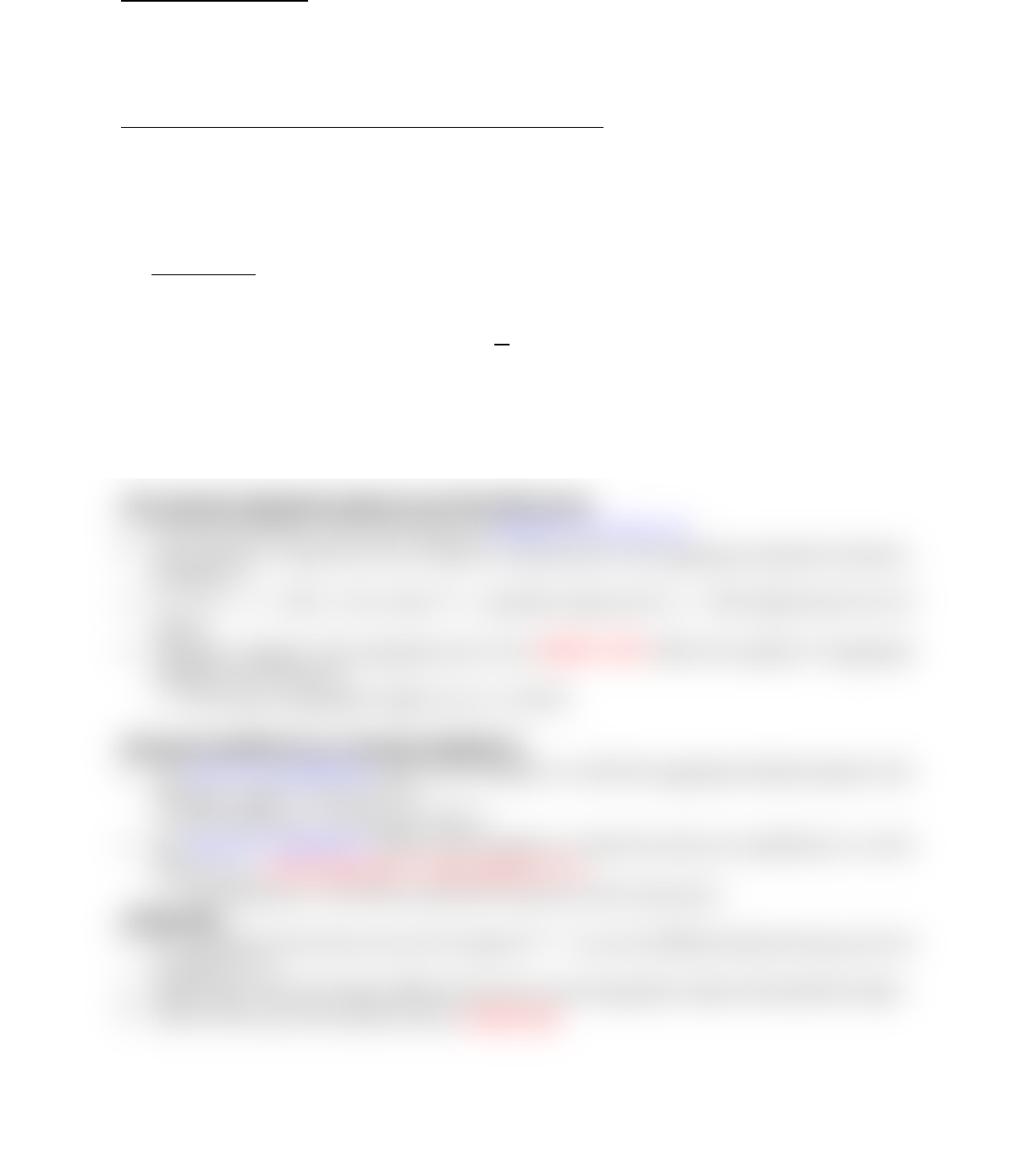# MGEB06H3 Lecture Notes - Lecture 6: Price Level, Aggregate Supply, Output Gap

18 views2 pagesMacroeconomics Notes: Lecture Six/Seven (Chapter Twelve) Part 2:
Aggregate Supply (AS)
The aggregate supply (AS) curve represents the quantity of output that producers are willing
to supply at each aggregate price level.
There are two types of aggregate supply one for the short run and one for the long run.
The Short-Run Aggregate Supply Curve (The SRAS Curve):
In macroeconomics, prices and wages are sticky in the short run. In particular, they are sticky
downward.
Nominal wages is the dollar amount of the wage paid.
Sticky wages are nominal wages that are slow to fall even in the face of high unemployed and
slow to rise even in the face of labour.
Assumption: Holding all else constant, producers are willing to supply more goods and
services in the short run when the aggregate price increases.
The short-run aggregate supply (SRAS) curve is upward sloping.
In generic form: SRAS: P = a + bYW
W
̅
̅
̅
,
Factors that affect producers’ willingness to supply other than Y & P will shift the SRAS
curve.
Producers’ willingness to supply is determined by their per-unit profit:
=Per-unit profit = Per-unit output price Per-unit production cost.
The Long-Run Aggregate Supply Curve (The LRAS Curve)
In macroeconomics, prices and wages are flexible in the long run.
The economy’s long-run level of output is determined by the aggregate production function.
(Chapter 9)
YP = YFE = A F(K, L, H), where YP = potential output and YFE = full-employment level of
output
Therefore, change in the aggregate price level DOES NOT affect the quantity of aggregate
supplied in the long run.
The long-run aggregate supply curve is vertical.
Short-Run Equilibrium vs. Long-Run Equilibrium
The short-run equilibrium refers to the situation in which the aggregate demand equals to the
aggregate supply in the short run.
The long-run equilibrium refers to the situation in which the short-run equilibrium is on the
LRAS curve. In the long run, Y must equal to YFE.
LR equilibrium: AD, SRAS and LRAS intersect at the same point.
Output Gap
It is possible for the short-run level of output (Y* = YSR) to be different than the long-run level
of output (YFE).
Output gap is the percentage differene between acyual aggregate output and potential output.
If this is the case, the economy has an output gap.
find more resources at oneclass.com
find more resources at oneclass.com
Unlock document

This preview shows half of the first page of the document.
Unlock all 2 pages and 3 million more documents.

# Get access

\$10 USD/m
Billed \$120 USD annually
Homework Help
Class Notes
Textbook Notes